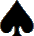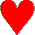Loreburn Bridge ClubRelease 2.19m
Bulletin
 Next Meeting of Loreburn Bridge Club Committee Tuesday 11th June 4:30pm
Recent Updates
 display_page2623rd May 2019 23:56 BSTHome Page21st May 2019 21:53 BST
 0 0 0 0 0 0
Pages viewed in 2019
Match Points Explained

Match Points Explained          Why Match Points??

Match points gives every board an equal value. I.e. a top score of 7NT has the same value as a top score of 2C (on another board).

The N/S and the E/W scores always complement each other. i.e. on any board if N/S get the highest score E/W gets the lowest score and vice versa.

To make this easier to understand I have used results from Wed 18th April.

The highest score per board is worked out by taking the number of tables subtract 1 and  then double the answer.

So for wed 16th April we had 10 tables. So 10-1= 9   then 9x2=18.

Hence maximum score per board for both N/S and E/W is 18.

 Position 1 2 3 4 5 6 7 8 9 10 Points 18 16 14 12 10 8 6 4 2 0

If 2  or more people have the same score then the points  available are shared.

1. .  Using Board 6

All the scores were made by W

Now 4 people scored 710  so the top 4 position scores are added and divided by 4

i.e. 18+16+14+12=60    then 60/4 =15

So Pair 19, Pair 11, Pair 20 and Pair 12 each receive 15 match points.

The next position is 5th and that score is 680 points.

So positions 5, 6, 7, 8   share 10, 8, 6, and 4 points

i.e.   10+8+6+4=28      then 28/4=7

so Pair 13, Pair15, Pair18 and Pair 14 each score 7 match points.

Now we are at position 9 and the next highest score is 650. Hence Pair 17 is awarded 2 match points.

Position 10 scores 500 (the lowest score) and so Pair 16 have 0 match points.

To find the N/S scores for each pair the E/W scores are subtracted from the maximum score of 18.

Hence Pair 19 E/W scored 15 hence N/S Pair 1 scored 3 match points

Pair 13 E/W scored 7 hence N/S Pair 3 scored 11 match points.

Pair 16 E/W scored 0 hence N/S Pair 10 scored 18.

**Simply put, for each board you get 2 Match Points for every pair you beat and one for every pair you tie with**

Now you need to find your Percentage for the evening.

1) Multiply the /number of tables by the top score per board i.e. 18 (max score)x 24(boards) =432

2) Add up all your points.

3) The percentage is your total match points/432 then Multiply by 100.

So for example pair 14 Total match points =241

Pair 14  Percentage is 241/432=0.5579   then 0.5579 x 100 =55.79%

Note. This is before Handicaps are added.

The good news is the computer does all the work.

Please ask me any questions on any part of this  you don’t understand and I’ll  try andexplain it to you.

Sandra Schneider